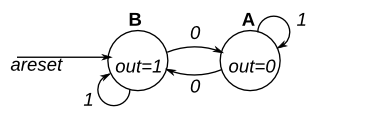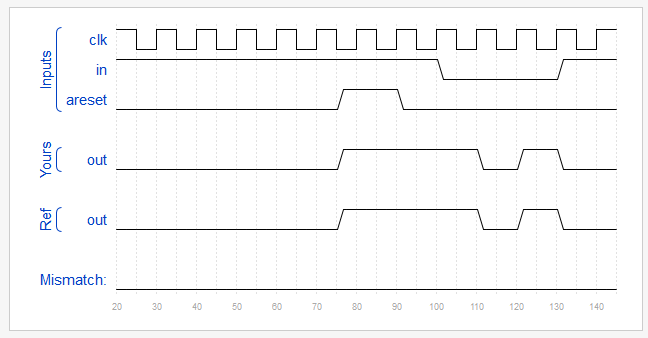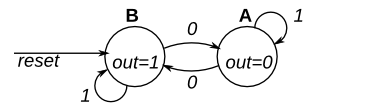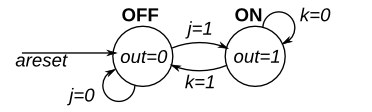# HDLBits 系列（24）进入FSM（有限状态机）的世界入口

Fsm1

Fsm1s

Fsm2

Fsm3comb

## Fsm1

This is a Moore state machine with two states, one input, and one output. Implement this state machine. Notice that the reset state is B.

This exercise is the same as fsm1s, but using asynchronous reset.Module Declaration

module top_module(
input clk,
input areset,    // Asynchronous reset to state B
input in,
output out);

module top_module(
input clk,
input areset,    // Asynchronous reset to state B
input in,
output out);//

parameter A=0, B=1;
reg state, next_state;

always @(posedge clk, posedge areset) begin    // This is a sequential always block
// State flip-flops with asynchronous reset
if(areset) state <= B;
else state <= next_state;
end

always @(*) begin    // This is a combinational always block
// State transition logic
case(state)
B: begin
if(in == 1) next_state = B;
else next_state = A;
end
A: begin
if(in == 1) next_state = A;
else next_state = B;
end
endcase
end

// Output logic
// assign out = (state == ...);
assign out = (state == A)?0:1;

endmodule## Fsm1s

This is a Moore state machine with two states, one input, and one output. Implement this state machine. Notice that the reset state is B.

This exercise is the same as fsm1, but using synchronous reset.Module Declaration

// Note the Verilog-1995 module declaration syntax here:
module top_module(clk, reset, in, out);
input clk;
input reset;    // Synchronous reset to state B
input in;
output out;

module top_module(
input clk,
input reset,    // Asynchronous reset to state B
input in,
output out);//

parameter A=0, B=1;
reg state, next_state;

always @(posedge clk) begin    // This is a sequential always block
// State flip-flops with asynchronous reset
if(reset) state <= B;
else state <= next_state;
end

always @(*) begin    // This is a combinational always block
// State transition logic
case(state)
B: begin
if(in == 1) next_state = B;
else next_state = A;
end
A: begin
if(in == 1) next_state = A;
else next_state = B;
end
endcase
end

// Output logic
// assign out = (state == ...);
assign out = (state == A)?0:1;

endmodule


## Fsm2

This is a Moore state machine with two states, two inputs, and one output. Implement this state machine.

This exercise is the same as fsm2s, but using asynchronous reset.Module Declaration

module top_module(
input clk,
input areset,    // Asynchronous reset to OFF
input j,
input k,
output out); 
module top_module(
input clk,
input areset,    // Asynchronous reset to OFF
input j,
input k,
output out); //

parameter OFF=0, ON=1;
reg state, next_state;

always @(*) begin
// State transition logic
case(state)
OFF: begin
if(j == 1) next_state = ON;
else next_state = OFF;
end
ON: begin
if(k == 1) next_state = OFF;
else next_state = ON;
end

endcase
end

always @(posedge clk, posedge areset) begin
// State flip-flops with asynchronous reset
if(areset) state <= OFF;
else state <= next_state;
end

// Output logic
// assign out = (state == ...);
assign out = (state == ON)? 1 : 0;

endmodule


## Fsm3comb

The following is the state transition table for a Moore state machine with one input, one output, and four states. Use the following state encoding: A=2'b00, B=2'b01, C=2'b10, D=2'b11.

Implement only the state transition logic and output logic (the combinational logic portion) for this state machine. Given the current state (state), compute the next_state and output (out) based on the state transition table.

StateNext stateOutput
in=0in=1
AAB0
BCB0
CAD0
DCB1

Module Declaration

module top_module(
input in,
input [1:0] state,
output [1:0] next_state,
output out); 

module top_module(
input in,
input [1:0] state,
output [1:0] next_state,
output out); //

parameter A=0, B=1, C=2, D=3;

// State transition logic: next_state = f(state, in)
always@(*) begin
case(state)
A: begin
if(in == 0) next_state = A;
else next_state = B;
end
B: begin
if(in == 0) next_state = C;
else next_state = B;
end
C: begin
if(in == 0) next_state = A;
else next_state = D;
end
D: begin
if(in == 0) next_state = C;
else next_state = B;
end
endcase
end

// Output logic:  out = f(state) for a Moore state machine
assign out = (state == D) ? 1:0;

endmodule


08-17
06-101万+08-106243
09-28201
04-092万+
05-17458
06-18400
07-261万+
02-13356
05-17107
11-2537
01-2116
12-151837
10-15164
07-26228
05-17821
08-1339
12-10747
05-25119
©️2020 CSDN 皮肤主题: 猿与汪的秘密 设计师:白松林